The branch node is a logical control family nodes provided in DataStudio. The branch node can define the Branch Logic and the direction of downstream branches under Different Logical Conditions.

## Create a branch node

The branch node is located in the Control class directory of the new node menu, as shown in the following figure.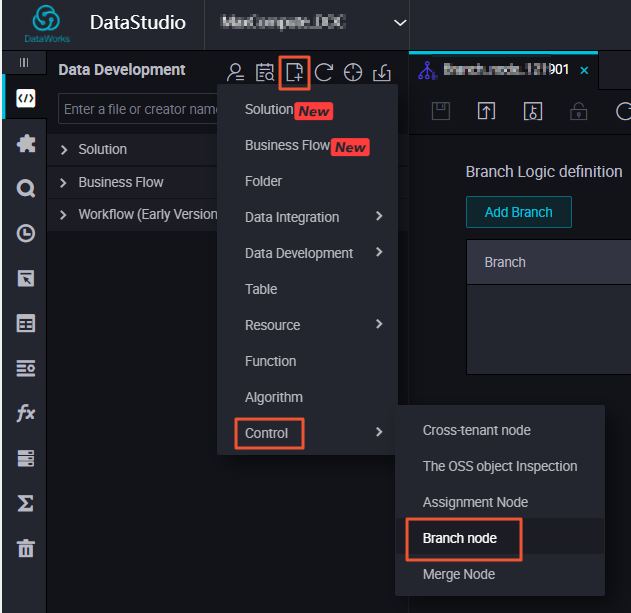## Define the branch logic

1. After creating the branch node, go to the Branch Logic Definition page, as shown in the following figure.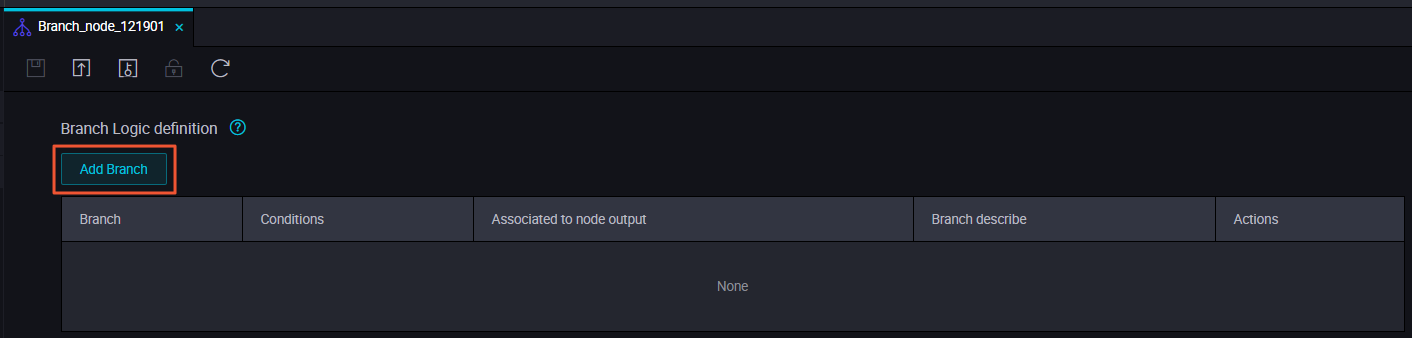2. In the Branch Logic Definition page, you can use Add Branch button to define the Branch Conditions, Associated to Node Output, and the Branch Describe, as shown in the following figure.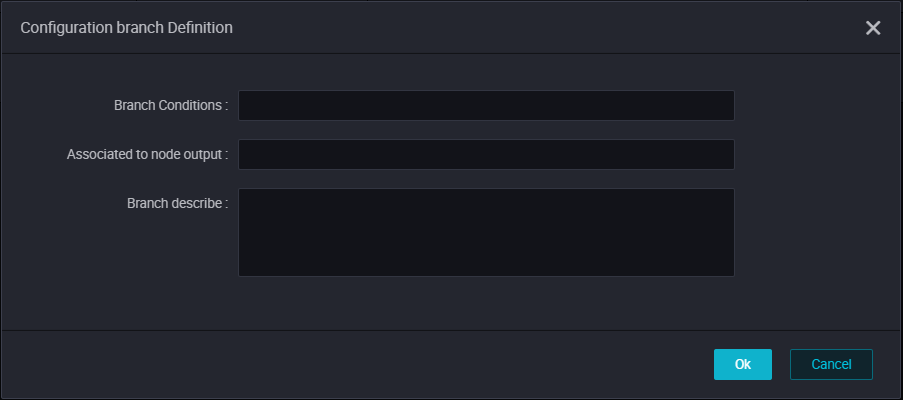The parameters are as follows:
• Branch conditions
• The branch condition only supports defining logical judgment condition according to Python comparison operators.
• If the value of the running state expression is true, it means the corresponding branching condition is satisfied. Otherwise, the branching condition is unsatisfactory.
• If a parsing error of the running state expression occurs, the running state of the whole branch node is set to failure.
• The branching conditions supports using global variables, and parameters defined in the node context, such as \${Input} in the figure. This can be a node input parameter defined in the branching node.
• Associated to node output
• The node output is used to mount dependencies for the downstream node of the branch node.
• When the branch does not meet conditions, the downstream node mounted on the associated node output is selected for running. This also refers to the status of other upstream nodes that the node depends on.
• When the branch does not meet the condition, the downstream node mounted on the associated node output will not run. The downstream node is placed in a not running state because it does not meet the branch condition.
• Branch description: The description of the branch definition.
Define two branches as follows: \${Input}==1 and \${Input}>2, as shown in the following figure.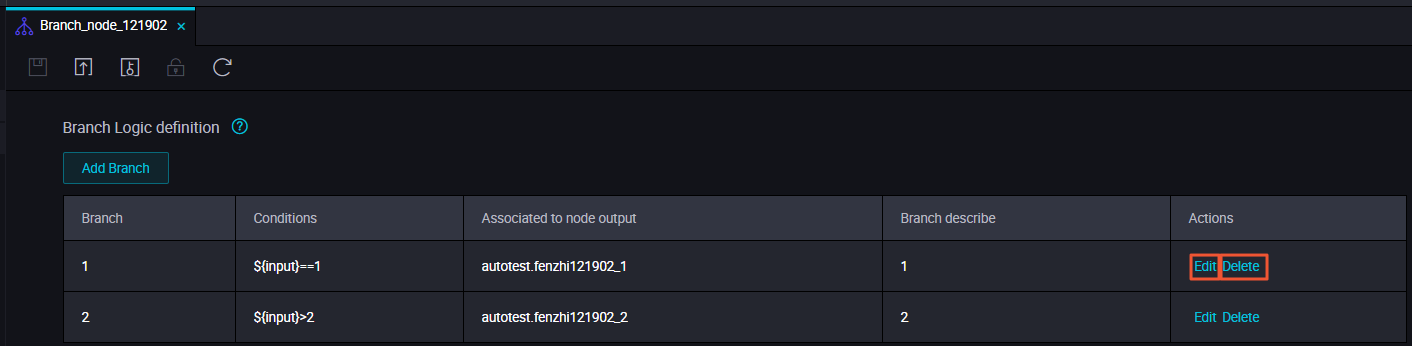• Edit: Click the Edit button to modify the setting branches. The related dependencies will also be updated.
• Delete: Click the Delete button to delete the setting branches. The related dependencies will also be updated.

## Scheduling configuration

After defining the branch condition, the output name is automatically added to the node Output of the Schedule, and the downstream node can depend on the output name mount. As shown in the following figure: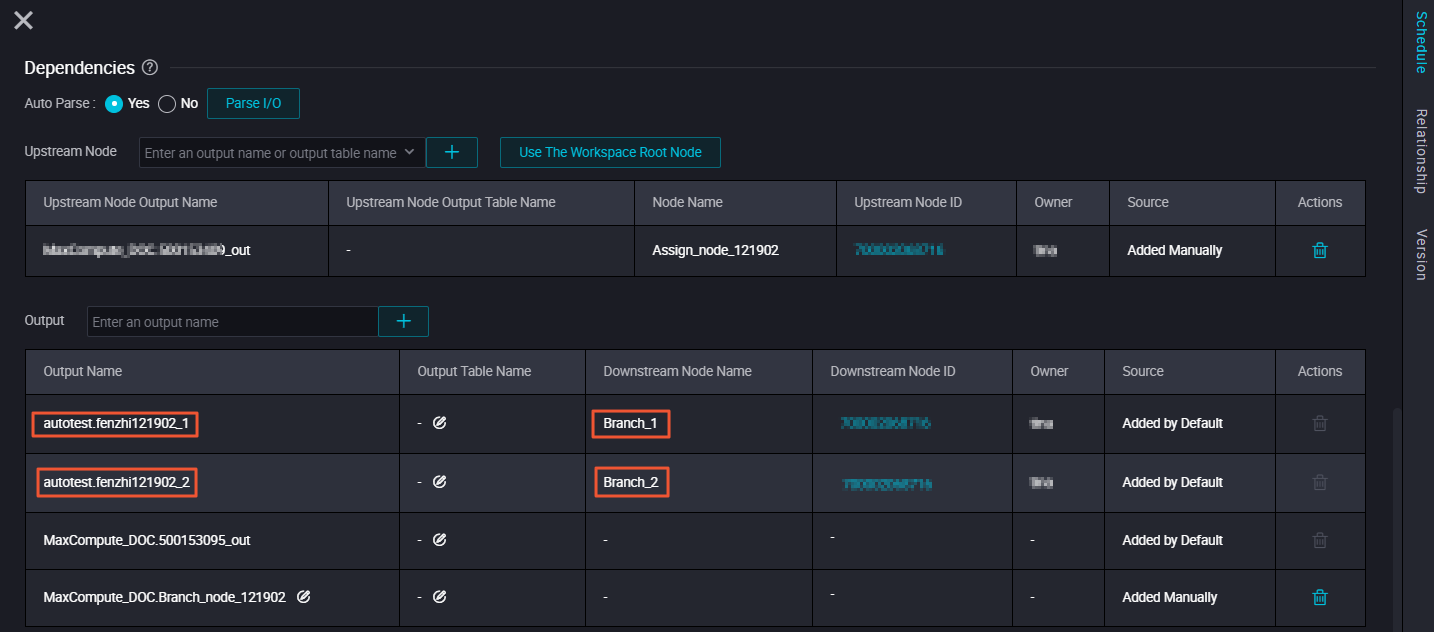Note You need to enter output records and context dependencies established by the connection manually if there are no output records in the scheduling configuration for context dependencies.

## Output case - downstream node mounted to a branch node

You can define the branch direction under different conditions by selecting the corresponding branch node output in the downstream node, after adding the branch node as the upstream node. For example, in the business process shown in the figure below, Branch_1 and Branch_2 are both downstream nodes of the branch node.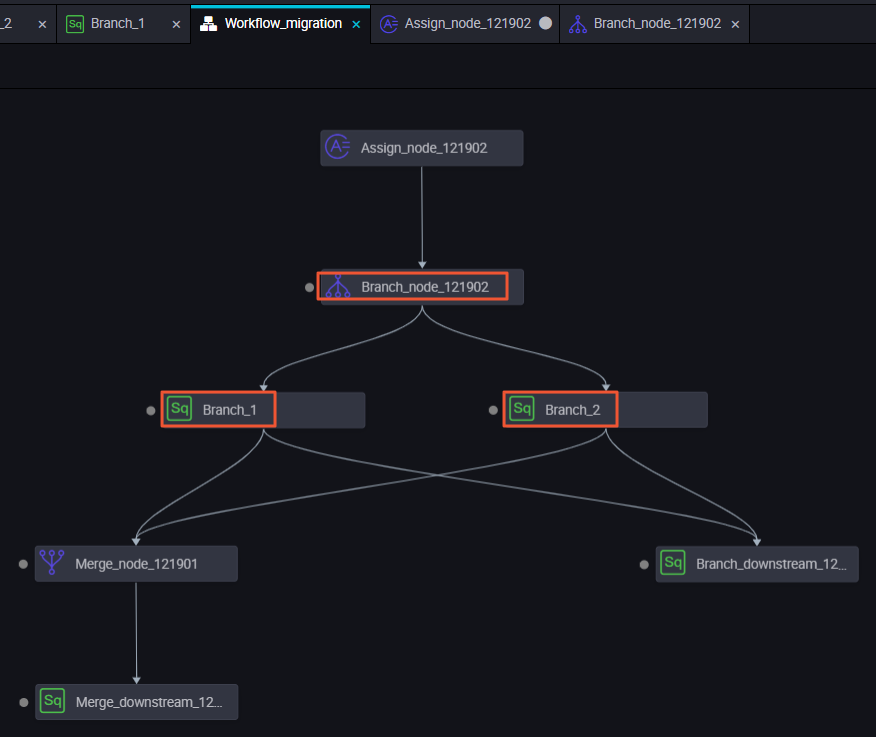Branch_1 depends on the output of 'autotest.fenzhi121902_1', as shown in the following figure.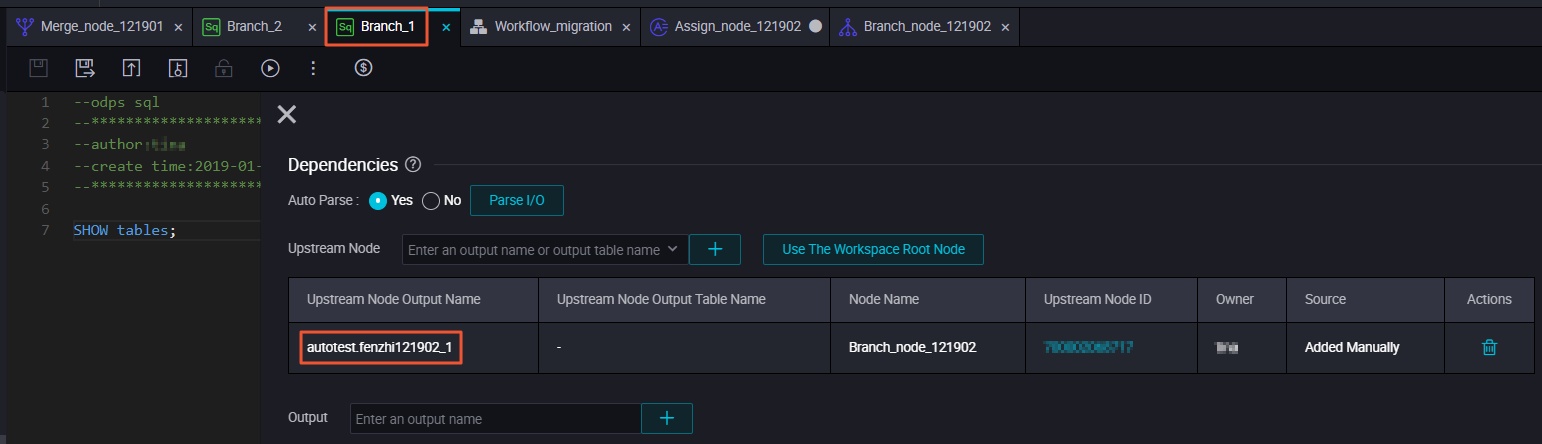Branch_2 depends on the output of 'autotest.fenzhi121902_2', as shown in the following figure.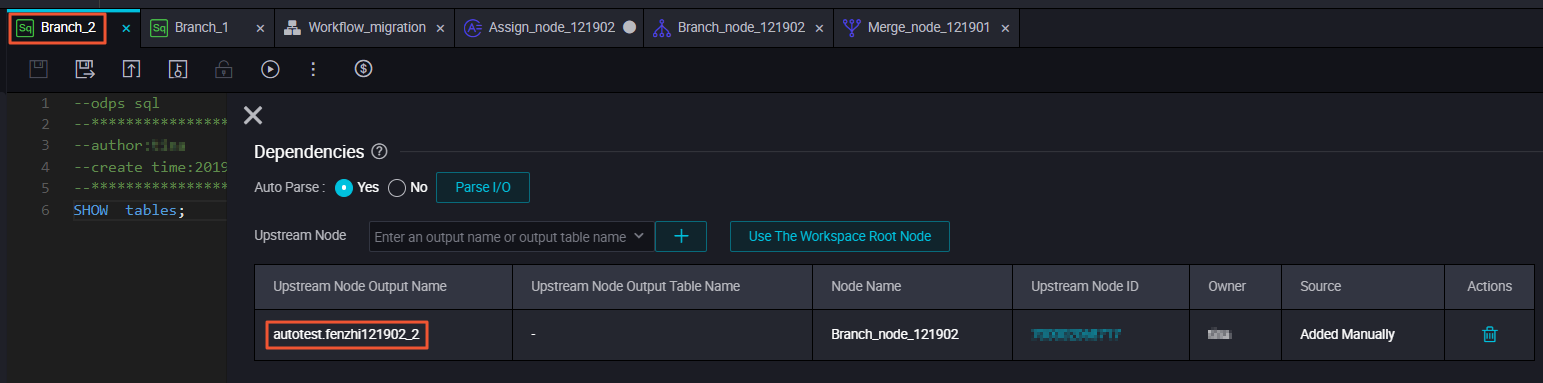## Submit scheduling operation

Submit the dispatch to the operation center to run, and the branch node satisfies the condition that is dependent on 'autotest.fenzhi121902_1' .Therefore, the print result of the log is as follows.
• When the branch meets the condition, select the downstream node of the branch to run. You can see the details of the run in Running Log.
• When the branch does not meet the condition, do not select the downstream node of the branch run. You can view the node set to 'skip' in the Running Log.

## Addition: supported Python comparison operators

In the following table, we assume that variable a is 10 and variable b is 20.

Comparison operators Description Example
== Equal - Compares objects for equality. （a==b）returns 'false'
!= Not equal - Compares whether two objects are not equal. （a!=b）returns 'true'
<> Not equal - Compares whether two objects are not equal. （a<>b）returns 'true'. This operator is similar to '!='.
> Greater than - Returns whether x is greater than y. （a>b）returns 'false'
< Less than - Returns whether x is less than y. All comparison operators return 1 for true, and 0 for false. This is equivalent to the special variables True and False, respectively. （a<b）returns 'true'
>= Greater than or equal to - Returns whether x is greater than or equal to y. （a>=b）returns 'false'
<= Less than or equal to - Returns whether x is less than or equal to y. （a<=b）returns 'true'